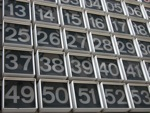0

## Quick Feats

Published on Thursday, February 28, 2013 in , ,In many of my posts, I tend to teach feats in great detail.

For a change of pace, today I'll be teaching 3 quick feats that can be learned fairly quickly.

LIGHT OR DARK CHESSBOARD SQUARES? Most chess games today are described in algebraic notation today. Given any square, A1 through H8, and without looking at a chessboard, can you tell me whether the given square is light or dark?

This is surprisingly easy once you get the approach down. First, instead of thinking of the squares as black and white, think of them as light and dark. This is important, as light has an odd number of letters, and dark has an even number of letters. This will be important later.

When given a coordinate in algebraic notation, ask yourself whether the letter is in the word CAGE. If the letter isn't in the word CAGE, you can ignore the letter completely, and just focus on the number part. If the letter is in the word CAGE, simply add 1 to the number, and then ignore the letter.

At this point, you'll have either an odd or even number. If the number is odd, then the given square is light (which has an odd number of letters). If the number is even, then the given square is dark (which has an even number of letters).

For example, is C7 light or dark? Since C is part of the word CAGE, we add 1 to the 7, giving us 8. 8 is an even number, so the square is dark. What about F3? F isn't in the word CAGE, so just ignore it. That leaves us with 3, which is an odd number, so the square is light.

You can have Wolfram|Alpha generate random practice squares for you, and use this diagram to check your answers.

SPEED OF A PASSING CAR? Here's a quick trick for mentally determining the speed of a passing car.

When you see in your rearview mirror that a car in another lane is about to pass you, keep driving at a steady speed, and quickly identify a landmark (lightpost, crosswalk, parking lot entrance, etc.) not too far ahead of you. When the car is even with you, begin counting at a steady rate, starting at 1. When the other car passes the landmark, remember the counting number (let's call this number a), and continue counting. When your car passes the same landmark, stop your count, and remember the final number in your count (we'll call this number b).

Once you have the numbers a and b, all you have to do is divide b by a, and then multiply that times your steady speed to get the speed of the other car.

For example, while driving at a steady speed of 35 mph yesterday, I saw a car coming up fast, and chose a lamppost up ahead as a landmark. When this car passed, I started counting one one-thousand, two one-thousand..., and noted that the other car reached the lamppost on a count of 5, while my car reached it at a count of 8. 8 ÷ 5 = 1.6, and 1.6 × 35 mph (my steady speed) = 56 mph (his speed), so I can estimate the other car was traveling about 56 miles per hour.

I originally ran across this bit of mental math on a now-defunct forum, and have found it to be very useful.

PRECISE SQUARE ROOTS OF NON-PERFECT SQUARES: This one is only quick to learn if you've already practiced and mastered my Estimating Square Roots feat. With just a minor alteration, you can actually give a more precise answer, using what are known as continued fractions.

The process starts the same as in the original feat, right up to the point where you determine the dividend by doubling the integer part of the fraction and then add 1. In this approach, you're going to stop the process before adding 1. You're then going to repeat that same fraction in the manner of a continued fraction.

To better explain this, let's try finding the square root of 261 using both approaches. In the estimation version, you would work out in your heard that the square root is approximately 16533. In this new version, you'll start writing a similar fraction, but don't add the 1. At first, all you would be writing in this new version is 16532 (note the 32 instead of the 33). Take the fractional part, 532, and add that same fraction to the bottom of the current fraction, and keep doing this over and over until you run out of room and/or your writing becomes too small. The result should look like this:

At this point, you can explain that this continued fraction, carried out to infinity, gives the exact square root of 261. Continued fractions are usually expressed with a 1 as each numerator, so the above approach is actually a generalized continued fraction.

With help from Gauss' notation for generalized continued fractions, it could also be entered into Wolfram|Alpha in this manner. Note that, when squared, this returns the exact given number!

This continued fraction approach is only the beginning! Over at Ted's Math World, Ted Muller explains how to create a wide variety of continued fractions for the same given number. If you prefer standard fractions, he also shows you how to create increasingly accurate fractions, as well.

That's enough quick feats for now. I hope you find them useful!